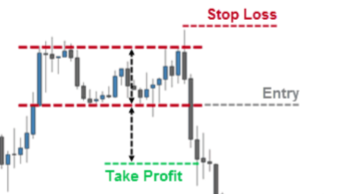Place the stop loss# Place the stop loss

By Boltvitaps | Trading Course | 5 May 2020

As we already know, stop losses are part of risk management and monetary management, aspects that we must understand very well in order to be profitable in the long term.

But where should Stop Loss be placed?

It should be placed where we think the price will never go.
So if it arrives, it takes our stop.
It means that in addition to being a stop loss tool to protect capital, it is also a confirmation of an error based on statistics.
We already know that in trading we will have a percentage of failed operations, caused by signals that did not work as expected.

For example, if you are trading the bounce on a resistance (we'll assume a double ceiling), the logical stop loss should be above that resistance (from the ceiling).

Why should the stop be above the ceiling?
Well, because if the price rises above, it was not a double roof.
Remember that we saw in the technical analysis that the patterns of the figures are not always met.

The level to cut losses should not be random, on the contrary, it should be based on technical analysis.
Some people do not know how to use it and therefore lose money constantly.

We will calculate the size of the position in this example so that it is satisfied that the stop is well placed (we assume a trader who invests 50% in a trade, although it is a lot, there are people who do it and we will take it as an example simply to understand the
subject of stop placement), with the following data from a trading account:

Total capital: u \$ s10,000

% of loss from operation (risk capital): 1%

% of capital in 1 single operation (capital invested): 50%

Placing the Stop Loss: To cut losses with this chart, the stop must be above the maximum (if they were to just bounce off the same level, it will be above that level).

The maximum is at US \$ 21.05, therefore we give it a few cents of margin and place the stop at US \$ 21.20.

Entry: We assume that we place a limited sell order (since we are short, downwards) near the close of the previous session, that is, the last green candle (or it could also be the maximum of the previous session) at \$ s20
, 82 and that our order is executed.

Transaction size: The account is \$ 10,000 and we will risk \$ 100 (1% of the account).
As the stop is at US \$ 21.20 and the entry at US \$ 20.82, the risk assumed is US \$ 0.38 per share sold.
Therefore, to determine the size of the operation you must first subtract the broker's commissions for both the entry and the exit (we will put \$ 10 as an example, it depends on the broker).
We would have \$ 90 left.
Since we are going to risk \$ 0.38 for each share, then we can sell 236 shares.
This calculation arises from making u \$ s90 / u \$ s0.38 = 236.84 and we round down the result.
Then the maximum position will be u \$ s5003.20 since in the event of a stop, we must buy them at that price, that is, we sold 236 shares at \$ 20.82 and we bought them at \$ 21.20, leaving us a loss of \$ 89
, 68.
But if the position is winning, assuming our target at \$ 18.03, we will have done the following: we sold 236 shares for \$ 20.82 and we bought them for \$ 18.03, that is, we sold \$ 4913.52 and bought \$
s4255.08 leaving us profit of \$ s658.44.

As you will notice, the risk is controlled, since in case of loss we have US \$ 89.68 (the US \$ 90 that remained, but it gives a few pennies less because we round the number of shares) plus the US \$ 10 of commissionsBoltvitaps

We know that it is a path that we want to travel together and to be able to answer the doubts that arise in this is part of our objective. In this line we share articles that give us their knowledge regarding "cryptocurrencies".